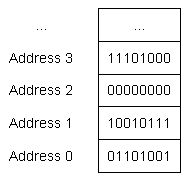# 4.1 — Introduction to fundamental data types

Bits, bytes, and memory addressing

In lesson 1.3 -- Introduction to variables, we talked about the fact that variables are names for a piece of memory that can be used to store information. To recap briefly, computers have random access memory (RAM) that is available for programs to use. When a variable is defined, a piece of that memory is set aside for that variable.

The smallest unit of memory is a binary digit (also called a bit), which can hold a value of 0 or 1. You can think of a bit as being like a traditional light switch -- either the light is off (0), or it is on (1). There is no in-between. If you were to look at a random segment of memory, all you would see is …011010100101010… or some combination thereof.

Memory is organized into sequential units called memory addresses (or addresses for short). Similar to how a street address can be used to find a given house on a street, the memory address allows us to find and access the contents of memory at a particular location.

Perhaps surprisingly, in modern computer architectures, each bit does not get its own unique memory address. This is because the number of memory addresses are limited, and the need to access data bit-by-bit is rare. Instead, each memory address holds 1 byte of data. A byte is a group of bits that are operated on as a unit. The modern standard is that a byte is comprised of 8 sequential bits.

Key insight

In C++, we typically work with “byte-sized” chunks of data.

The following picture shows some sequential memory addresses, along with the corresponding byte of data:As an aside...

Some older or non-standard machines may have bytes of a different size (from 1 to 48 bits) -- however, we generally need not worry about these, as the modern de-facto standard is that a byte is 8 bits. For these tutorials, we’ll assume a byte is 8 bits.

Data types

Because all data on a computer is just a sequence of bits, we use a data type (often called a “type” for short) to tell the compiler how to interpret the contents of memory in some meaningful way. You have already seen one example of a data type: the integer. When we declare a variable as an integer, we are telling the compiler “the piece of memory that this variable uses is going to be interpreted as an integer value”.

When you give an object a value, the compiler and CPU take care of encoding your value into the appropriate sequence of bits for that data type, which are then stored in memory (remember: memory can only store bits). For example, if you assign an integer object the value 65, that value is converted to the sequence of bits `0100 0001` and stored in the memory assigned to the object.

Conversely, when the object is evaluated to produce a value, that sequence of bits is reconstituted back into the original value. Meaning that `0100 0001` is converted back into the value 65.

Fortunately, the compiler and CPU do all the hard work here, so you generally don’t need to worry about how values gets converted into bit sequences and back.

All you need to do is pick a data type for your object that best matches your desired use.

Fundamental data types

C++ comes with built-in support for many different data types. These are called fundamental data types, but are often informally called basic types, primitive types, or built-in types.

Here is a list of the fundamental data types, some of which you have already seen:

Types Category Meaning Example
float
double
long double
Floating Point a number with a fractional part 3.14159
bool Integral (Boolean) true or false true
char
wchar_t
char8_t (C++20)
char16_t (C++11)
char32_t (C++11)
Integral (Character) a single character of text ‘c’
short
int
long
long long (C++11)
Integral (Integer) positive and negative whole numbers, including 0 64
std::nullptr_t (C++11) Null Pointer a null pointer nullptr
void Void no type n/a

This chapter is dedicated to exploring these fundamental data types in detail (except std::nullptr_t, which we’ll discuss when we talk about pointers). C++ also supports a number of other more complex types, called compound types. We’ll explore compound types in a future chapter.

Author's note

The terms `integer` and `integral` are similar, but have different meanings. An `integer` is a specific data type that hold non-factional numbers, such as whole numbers, 0, and negative whole numbers. `Integral` means “like an integer”. Most often, `integral` is used as part of the term `integral type`, which includes all of the Boolean, characters, and integer types (also enumerated types, which we’ll discuss in chapter 8). `Integral type` are named so because they are stored in memory as integers, even though their behaviors might vary (which we’ll see later in this chapter when we talk about the character types).

The _t suffix

Many of the types defined in newer versions of C++ (e.g. std::nullptr_t) use a _t suffix. This suffix means “type”, and it’s a common nomenclature applied to modern types.

If you see something with a _t suffix, it’s probably a type. But many types don’t have a _t suffix, so this isn’t consistently applied.4.2 -- VoidIndex3.x -- Chapter 3 summary and quiz

### 241 comments to 4.1 — Introduction to fundamental data types

•random

Typos police

•bert

You may have a couple of typos:
so you generally don’t need to worry about how values gets converted into bit sequences and back.
gets => get

and
An integer is a specific data type that hold non-factional numbers, such as whole numbers, 0, and negative whole numbers.
non-factional => non-fractional

Thank you for such a wonderful resource!